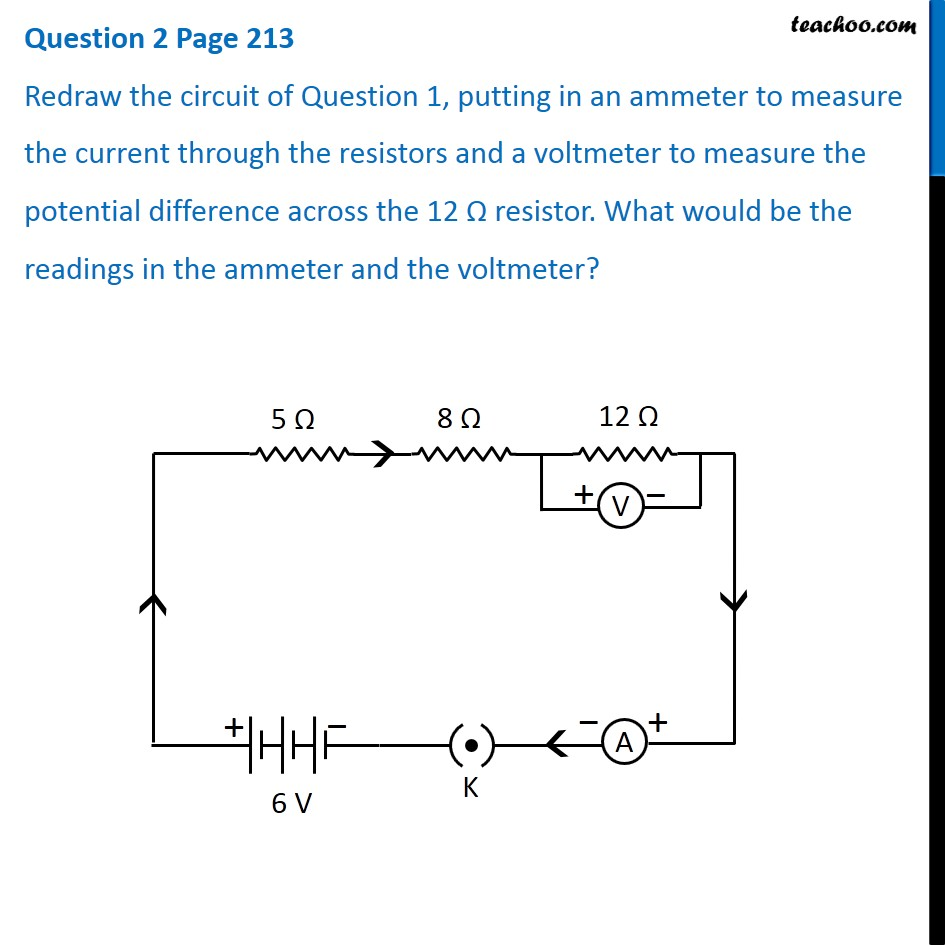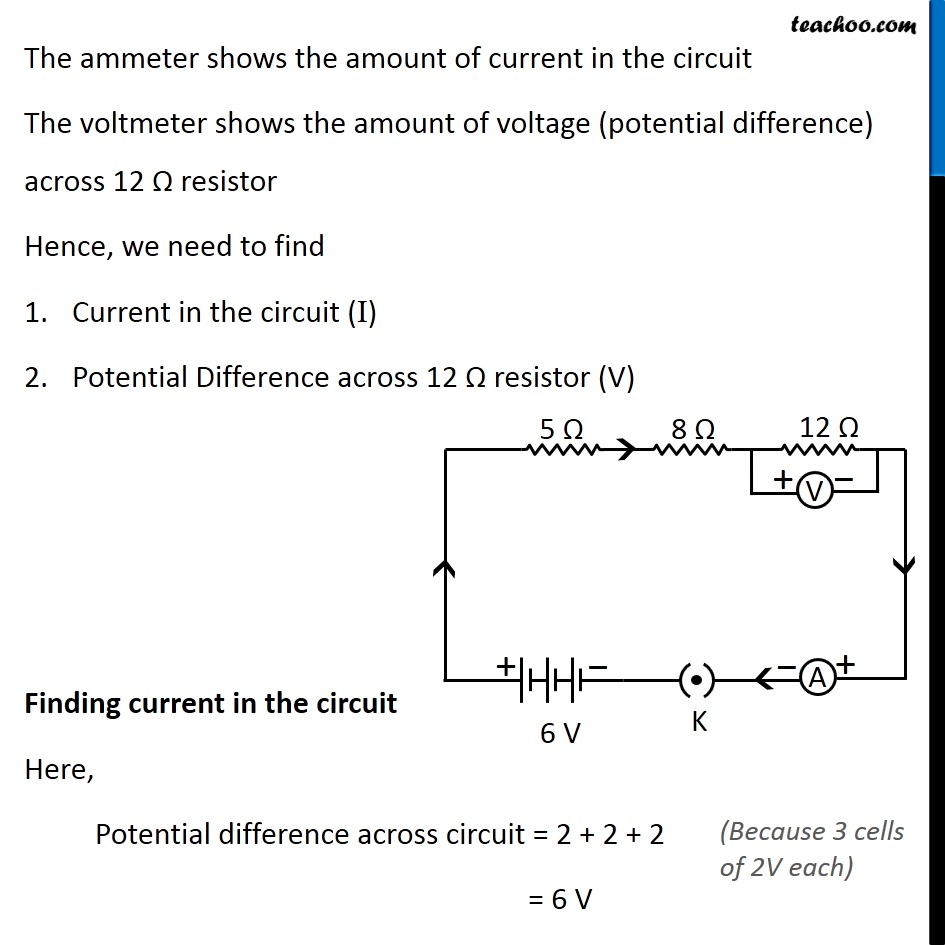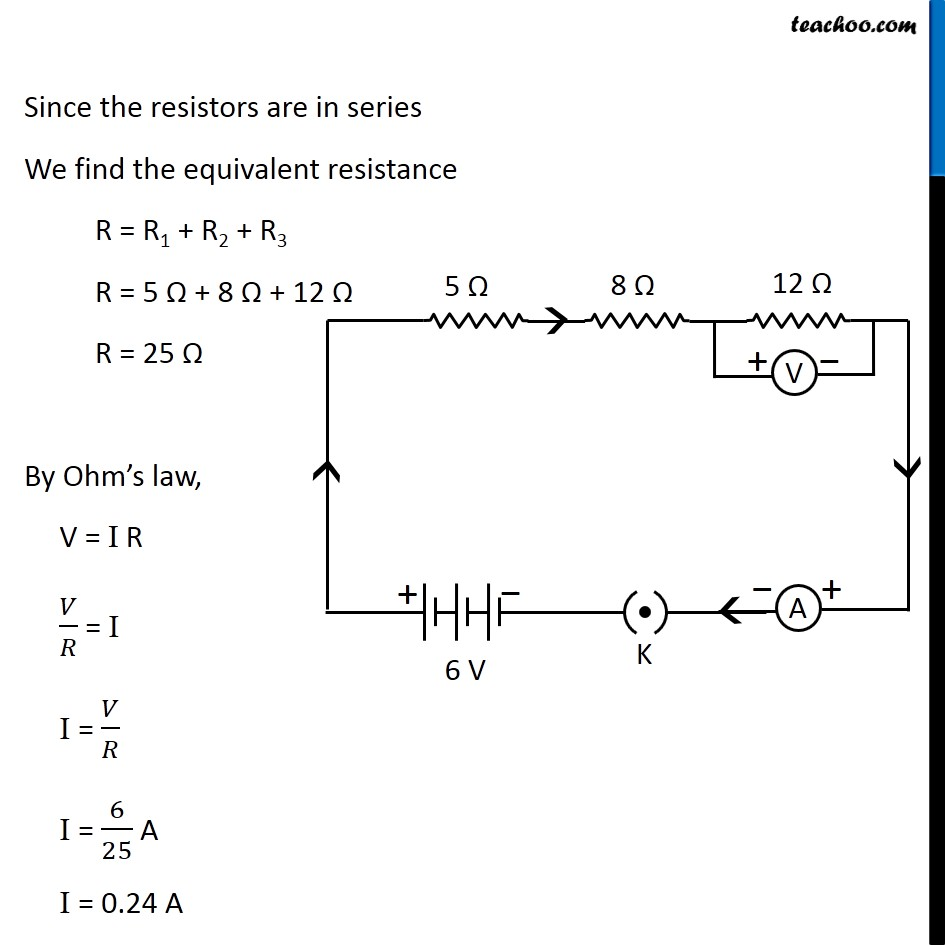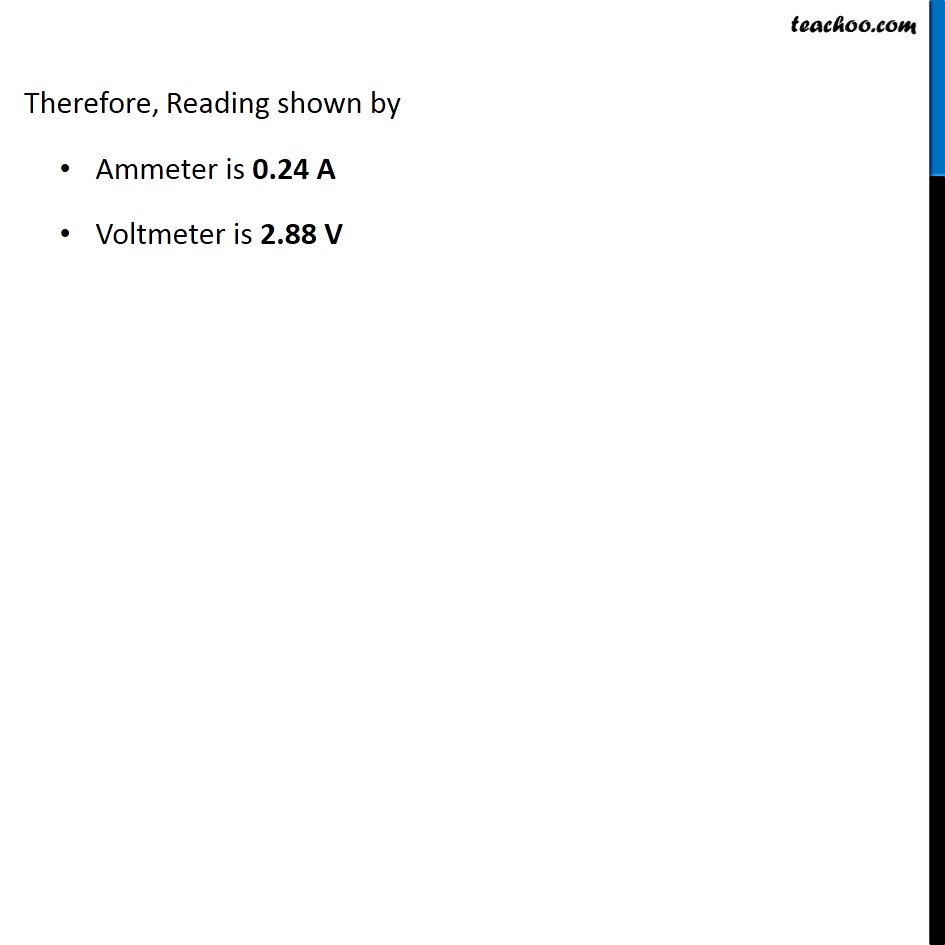Questions from Inside the chapter

Class 10
Chapter 12 Class 10 - Electricity

## Redraw the circuit of Question 1, putting in an ammeter to measure the current through the resistors and a voltmeter to measure the potential difference across the 12 Ω resistor. What would be the readings in the ammeter and the voltmeter?Learn in your speed, with individual attention - Teachoo Maths 1-on-1 Class

### Transcript

Question 2 Page 213 Redraw the circuit of Question 1, putting in an ammeter to measure the current through the resistors and a voltmeter to measure the potential difference across the 12 Ω resistor. What would be the readings in the ammeter and the voltmeter? The ammeter shows the amount of current in the circuit The voltmeter shows the amount of voltage (potential difference) across 12 Ω resistor Hence, we need to find Current in the circuit (I) Potential Difference across 12 Ω resistor (V) Finding current in the circuit Here, Potential difference across circuit = 2 + 2 + 2 = 6 V (Because 3 cells of 2V each) Since the resistors are in series We find the equivalent resistance R = R1 + R2 + R3 R = 5 Ω + 8 Ω + 12 Ω R = 25 Ω By Ohm’s law, V = I R 𝑉/𝑅 = I I = 𝑉/𝑅 I = 6/25 A I = 0.24 A Finding potential difference across 12 Ω resistor In series combination, current flowing through all resistors is same ∴ I = 6/25 A R = 12 Ω By Ohm’s law, V = I R V = 6/25 × 12 V = 72/25 V = 2.88 V Therefore, Reading shown by Ammeter is 0.24 A Voltmeter is 2.88 V